# Find the inverse of the matrix (if it exists). (If an answer does not exist, enter...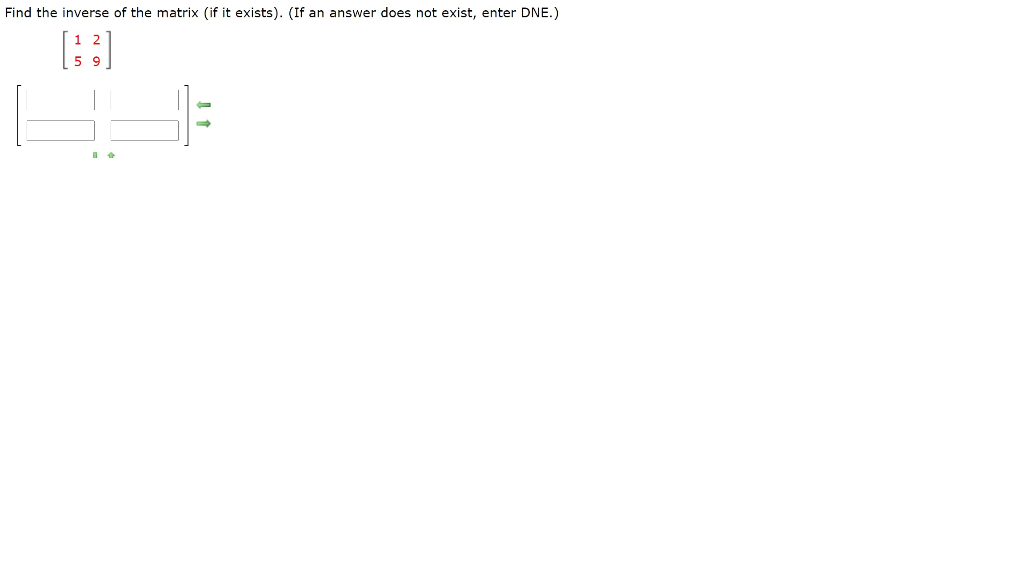Find the inverse of the matrix (if it exists). (If an answer does not exist, enter DNE.)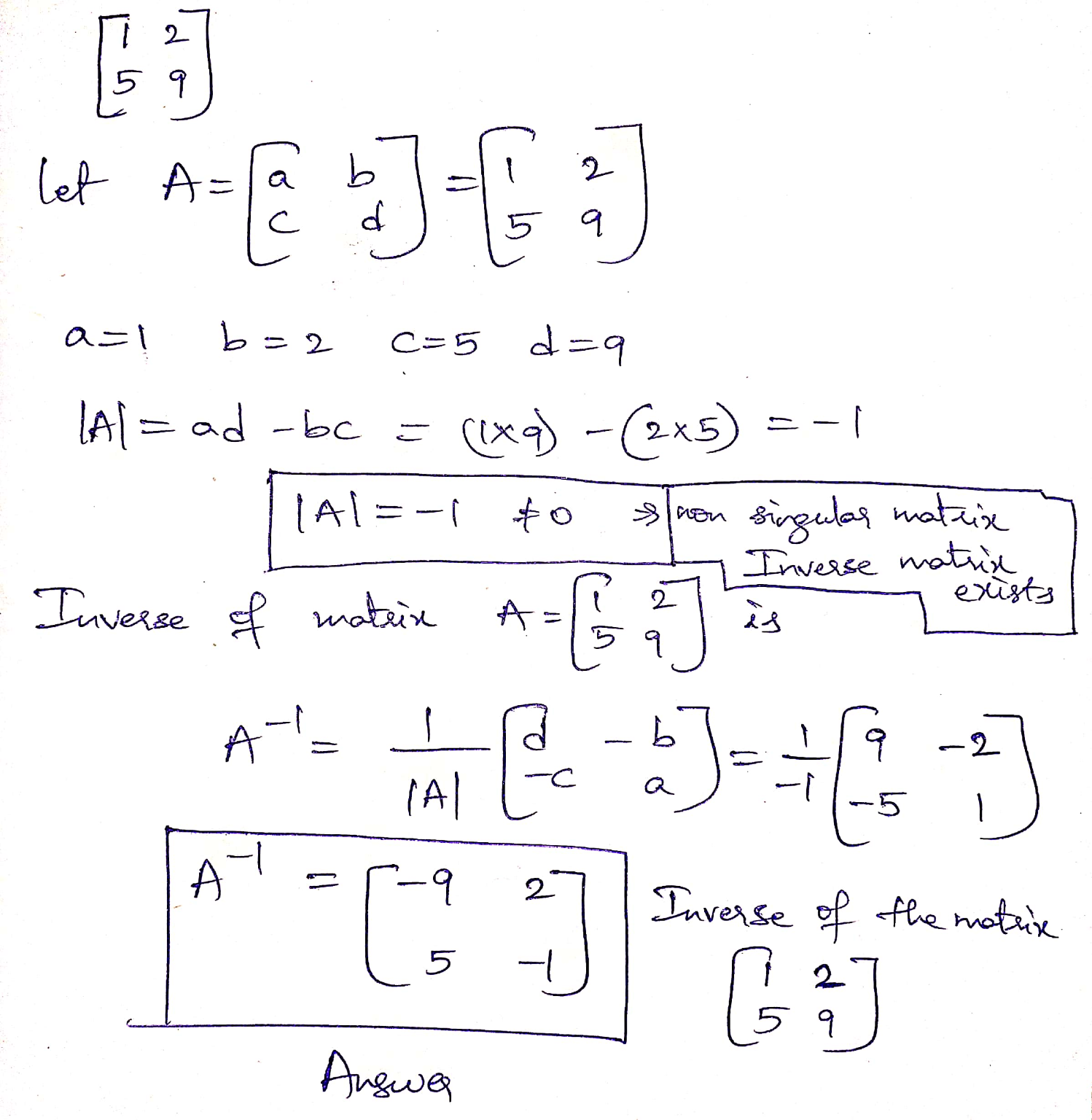#### Earn Coin

Coins can be redeemed for fabulous gifts.

Similar Homework Help Questions
• ### Find the inverse of the matrix (if it exists). (If an answer does not exist, enter...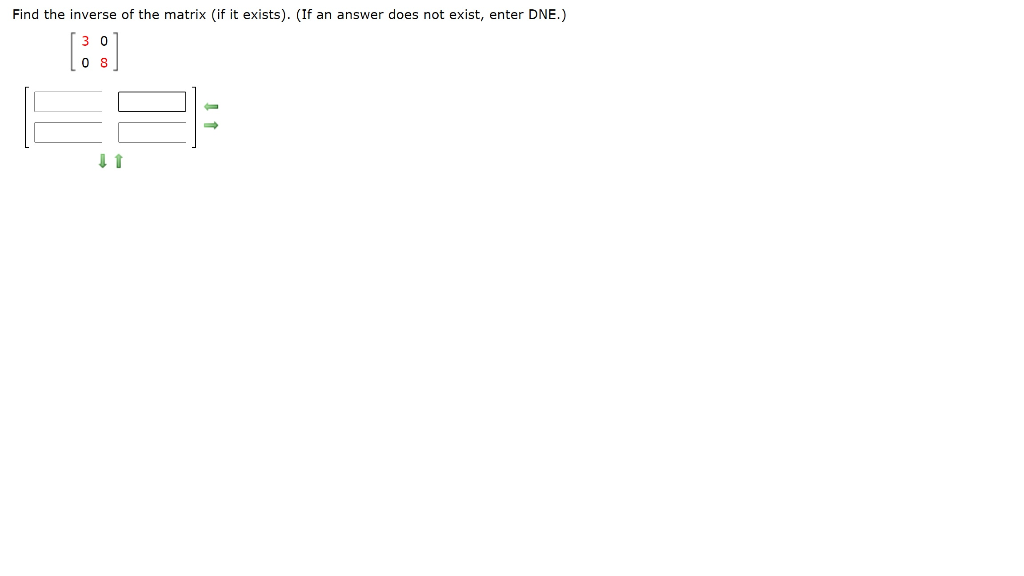Find the inverse of the matrix (if it exists). (If an answer does not exist, enter DNE.) 08 1 Find the inverse of the matrix (if it exists). (If an answer does not exist, enter DNE.)

• ### Find the inverse of the matrix (if it exists). (If an answer does not exist, enter...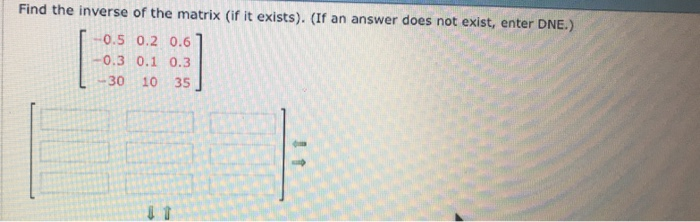Find the inverse of the matrix (if it exists). (If an answer does not exist, enter DNE.) 0.5 0.2 0.6 -0.3 0.1 0.3 L -30 10 35 J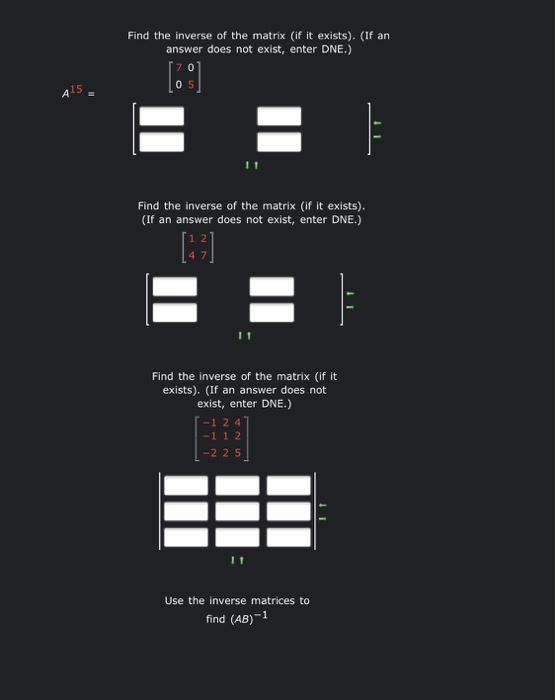3 part question about inverse of matrices. please help!! Find the inverse of the matrix (if it exists). (If an answer does not exist, enter DNE.)  05 415 E Find the inverse of the matrix (if it exists). (If an answer does not exist, enter DNE.) E = Find the inverse of the matrix (if it exists). (If an answer does not exist, enter DNE.) 1-1 2 4 -1 1 2 | -2 25 Use the inverse matrices to...

• ### Evaluate the limit, if it exists. (If an answer does not exist, enter DNE.) lim V...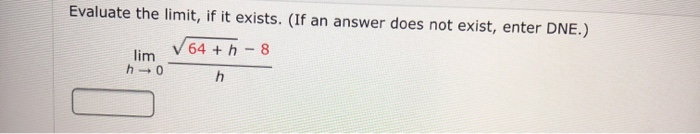Evaluate the limit, if it exists. (If an answer does not exist, enter DNE.) lim V 64 + - 8 h0

• ### Linear Algebra Use the Quick Formula to find A1, if it exists. (If the inverse does...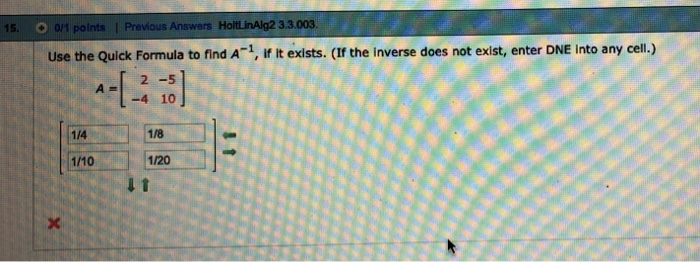Linear Algebra Use the Quick Formula to find A1, if it exists. (If the inverse does not exist, enter DNE Into any cell.) 2 -5 -4 10 1/4 1/8 1/10 1/20

• ### Use properties of limits and algebraic methods to find the limit, if it exists. (If the limit is infinite, enter '∞' or '-∞', as appropriate. If the limit does not otherwise exist, enter DNE.)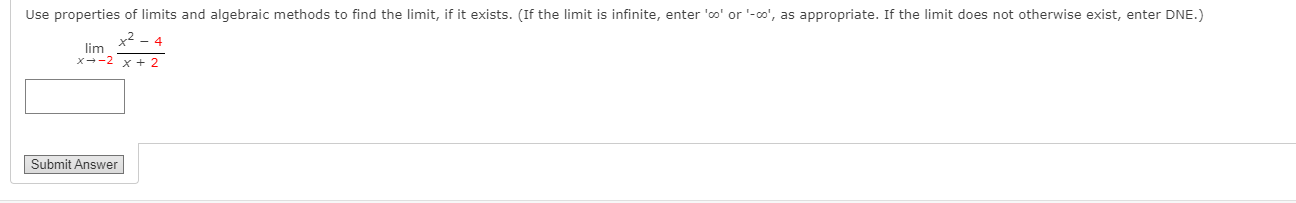Use properties of limits and algebraic methods to find the limit, if it exists. (If the limit is infinite, enter '∞' or '-∞', as appropriate. If the limit does not otherwise exist, enter DNE.)

• ### Use properties of limits and algebraic methods to find the limit, if it exists. (If the limit is infinite, enter '∞' or '-∞', as appropriate. If the limit does not otherwise exist, enter DNE.)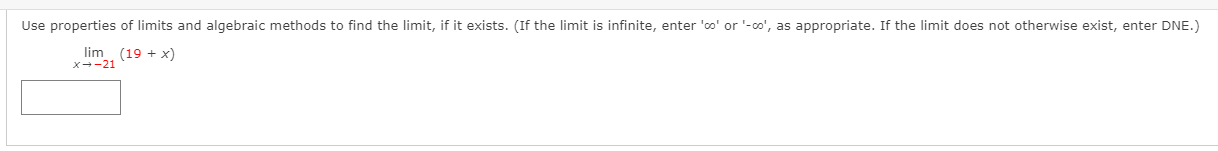Use properties of limits and algebraic methods to find the limit, if it exists. (If the limit is infinite, enter '∞' or '-∞', as appropriate. If the limit does not otherwise exist, enter DNE.)

• ### Use properties of limits and algebraic methods to find the limit, if it exists. (If the limit is infinite, enter '∞' or '-∞', as appropriate. If the limit does not otherwise exist, enter DNE.)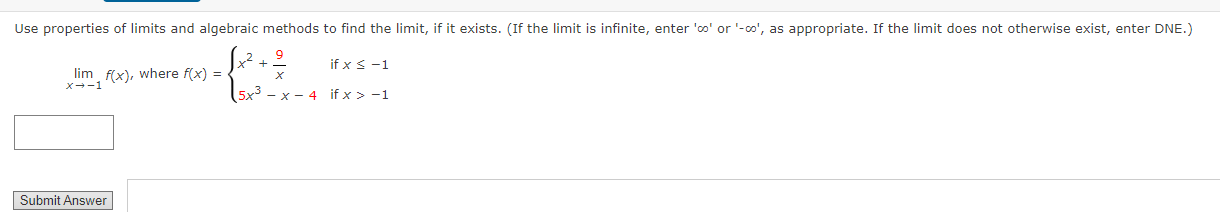Use properties of limits and algebraic methods to find the limit, if it exists. (If the limit is infinite, enter '∞' or '-∞', as appropriate. If the limit does not otherwise exist, enter DNE.)

• ### Use properties of limits and algebraic methods to find the limit, if it exists. (If the limit is infinite, enter '∞' or '-∞', as appropriate. If the limit does not otherwise exist, enter DNE.)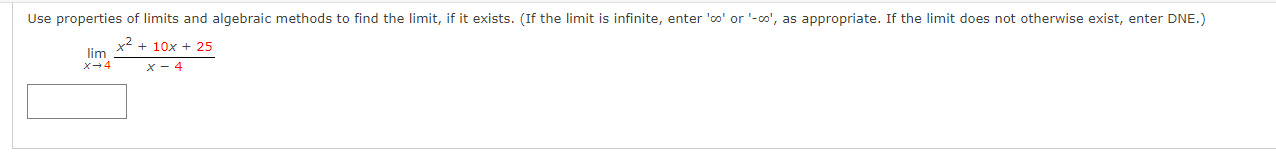Use properties of limits and algebraic methods to find the limit, if it exists. (If the limit is infinite, enter '∞' or '-∞', as appropriate. If the limit does not otherwise exist, enter DNE.)

• ### Use properties of limits and algebraic methods to find the limit, if it exists. (If the limit is infinite, enter '∞' or '-∞', as appropriate. If the limit does not otherwise exist, enter DNE.)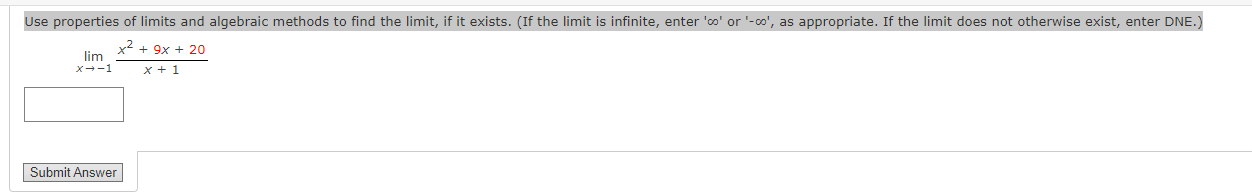Use properties of limits and algebraic methods to find the limit, if it exists. (If the limit is infinite, enter '∞' or '-∞', as appropriate. If the limit does not otherwise exist, enter DNE.)